# QUESTION 1 Please help me with my homework assignment. This is question 1 and 2. Thank...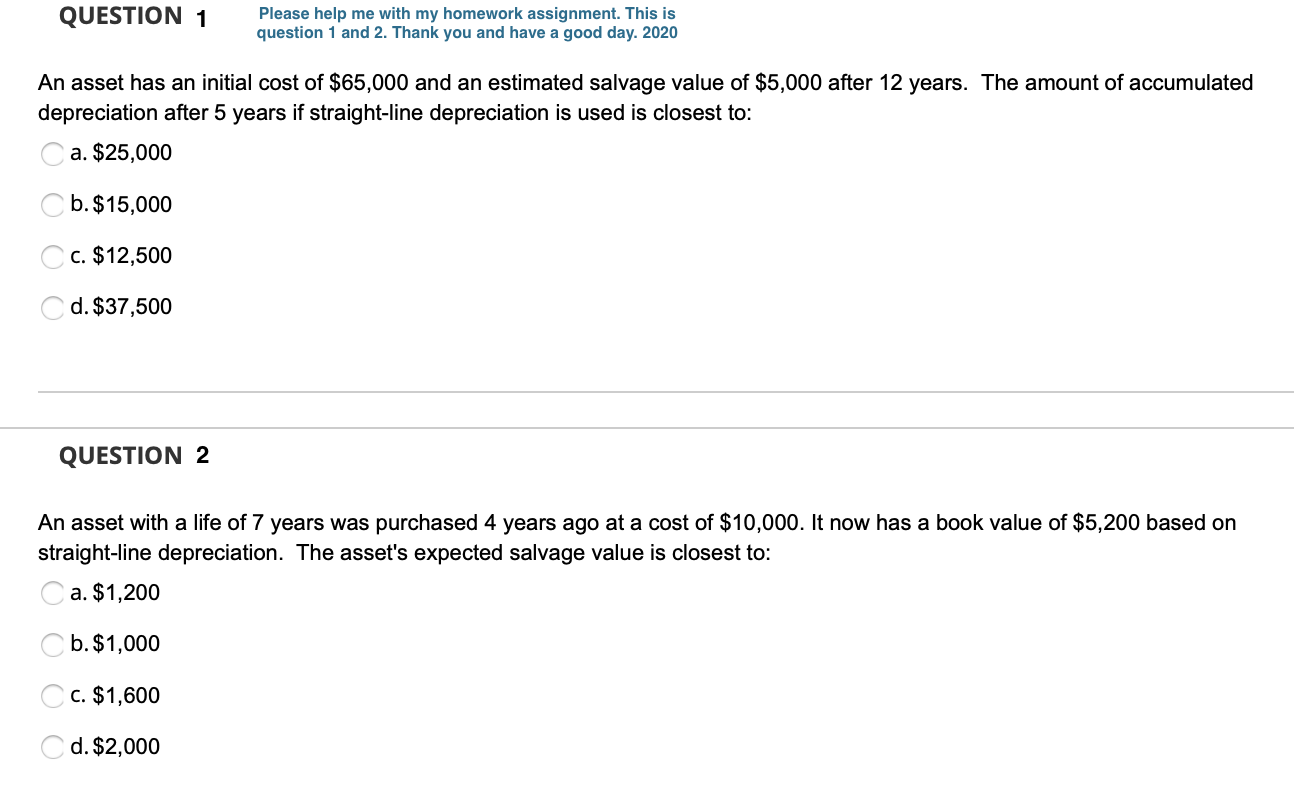QUESTION 1 Please help me with my homework assignment. This is question 1 and 2. Thank you and have a good day. 2020 An asset has an initial cost of \$65,000 and an estimated salvage value of \$5,000 after 12 years. The amount of accumulated depreciation after 5 years if straight-line depreciation is used is closest to: a. \$25,000 Cb. \$15,000 C. \$12,500 C d. \$37,500 QUESTION 2 An asset with a life of 7 years was purchased 4 years ago at a cost of \$10,000. It now has a book value of \$5,200 based on straight-line depreciation. The asset's expected salvage value is closest to: Ca. \$1,200 C b. \$1,000 C C. \$1,600 Cd. \$2,000

Question:1

The Answer is A. \$ 25,000

Working:

 Equipment Cost \$               65,000 Estimated Salvage Value \$                 5,000 Useful Life 12 Years Accumulated Depreciation after 5 Years [(65000-5000)/12Years*5Years] \$               25,000

So, Remaining options are incorrect.
Question:2

The Answer is C. \$ 1,600

Working:

 Equipment Cost \$               10,000 Book Value After 4 Years \$                 5,200 Useful Life 7 Years If Salvage Value is \$                 1,600 Book Value After 4 Years[10000-((10000-1600)/7Years*4Years)] \$                 5,200

So, Remaining options are incorrect.

#### Earn Coin

Coins can be redeemed for fabulous gifts.

Similar Homework Help Questions
• ### QUESTION 13 An asset with a life of 7 years was purchased 4 years ago at...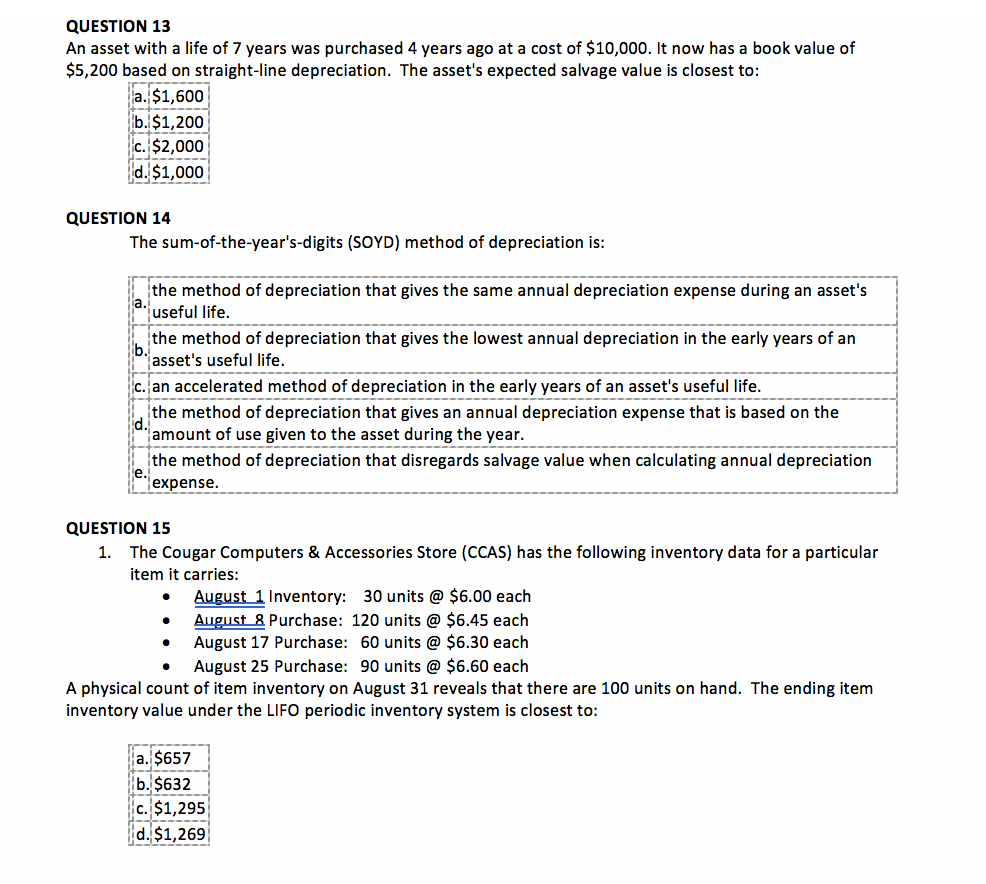QUESTION 13 An asset with a life of 7 years was purchased 4 years ago at a cost of \$10,000. It now has a book value of \$5,200 based on straight-line depreciation. The asset's expected salvage value is closest to: a. \$1,600 b. \$1,200 c. \$2,000 d. \$1,000 QUESTION 14 The sum-of-the-year's-digits (SOYD) method of depreciation is: a the method of depreciation that gives the same annual depreciation expense during an asset's useful life. the method of depreciation that gives...

• ### 9- An accrued expense amounting to \$18,000 was overlooked when ascertaining the profit for the year....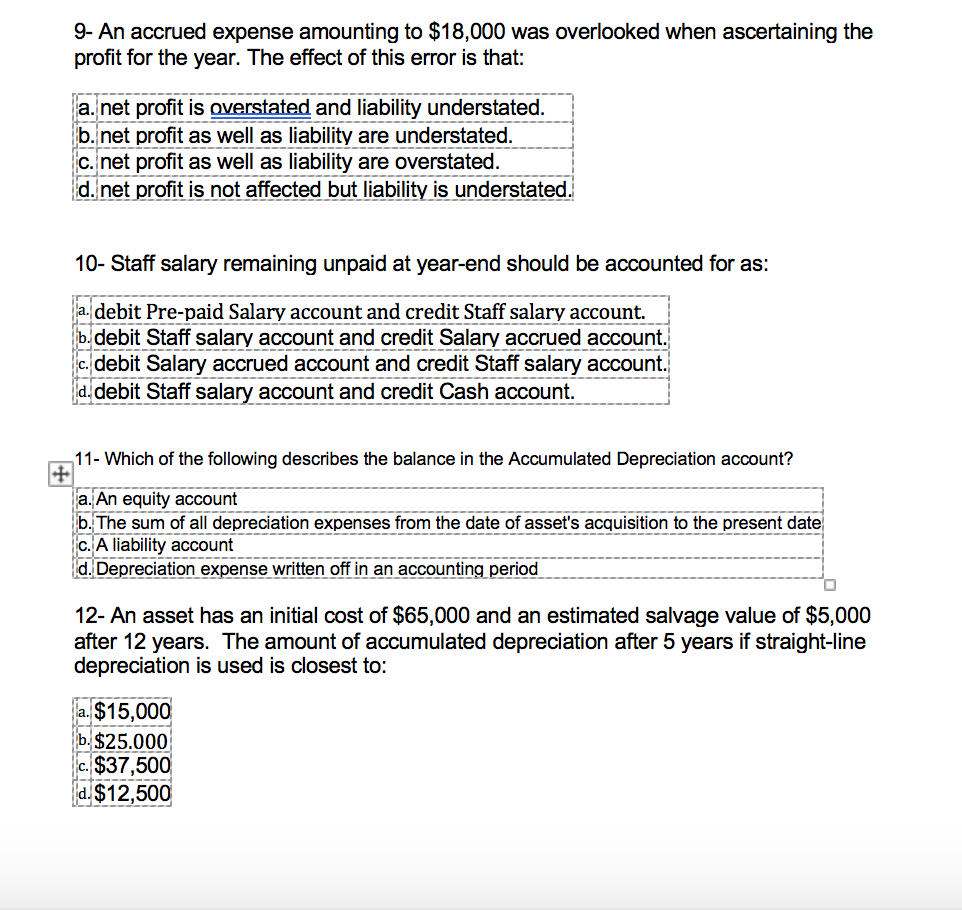9- An accrued expense amounting to \$18,000 was overlooked when ascertaining the profit for the year. The effect of this error is that: a. net profit is overstated and liability understated. b.net profit as well as liability are understated. c. net profit as well as liability are overstated. d. net profit is not affected but liability is understated. 10- Staff salary remaining unpaid at year-end should be accounted for as: a. debit Pre-paid Salary account and credit Staff salary account....

• ### P M Gmail Youtube Maps er 18 Homework Problem 18.2A Using different depreciation methods and comparing...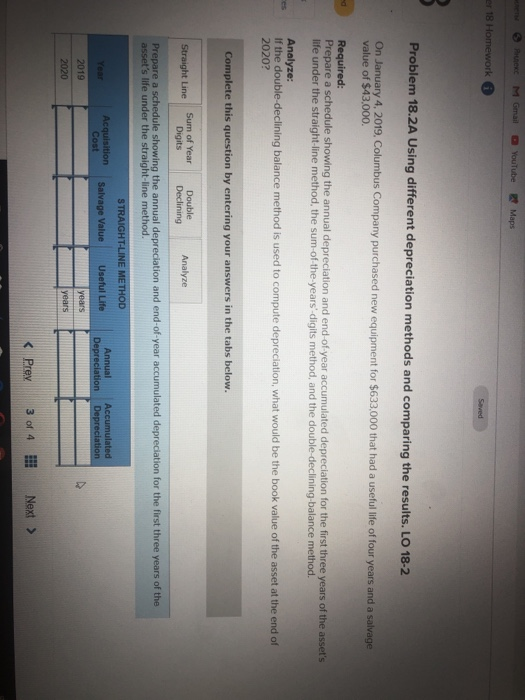P M Gmail Youtube Maps er 18 Homework Problem 18.2A Using different depreciation methods and comparing the results. LO 18-2 On January 4, 2019. Columbus Company purchased new equipment for \$633,000 that had a useful life of four years and a salvage value of \$43,000. Required: Prepare a schedule showing the annual depreciation and end-of-year accumulated depreciation for the first three years of the asset's life under the straight-line method, the sum-of-the-years' digits method, and the double-declining balance method. Analyze:...

• ### Check my work Exercise 18.4 Computing depreciation under various methods. LO 18-2 25 points M. Com...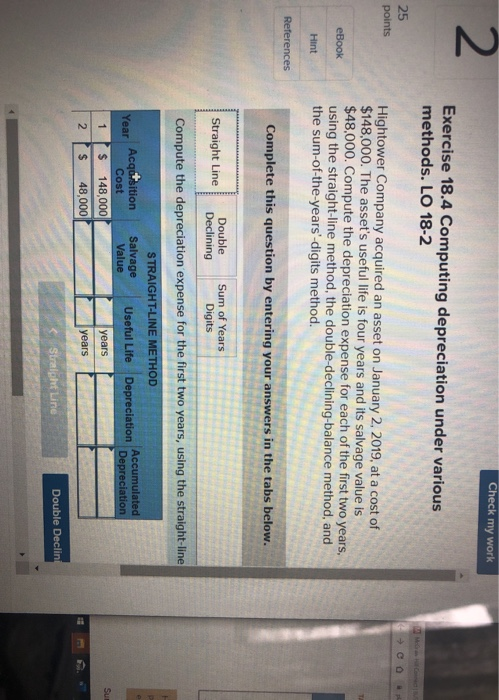Check my work Exercise 18.4 Computing depreciation under various methods. LO 18-2 25 points M. Com →CO Hightower Company acquired an asset on January 2, 2019, at a cost of \$148,000. The asset's useful life is four years and its salvage value is \$48,000. Compute the depreciation expense for each of the first two years, using the straight-line method, the double-declining-balance method, and the sum-of-the-years-digits method. eBook Hint References Complete this question by entering your answers in the tabs below....

• ### 1. Which of the following is not needed to calculate annual straight-line depreciation? a. fair value one year after acq...

1. Which of the following is not needed to calculate annual straight-line depreciation? a. fair value one year after acquisition b. cost of the asset c. estimated useful life d. estimated salvage value 2. Depreciation methods which take larger amounts of depreciation in the early years of an asset's useful life are referred to as a. accelerated b. aggressive c, cost based d. unauthorized

• ### Please check if it's correct. If not, please explain and re-do it. Thanks Assignment 2 O...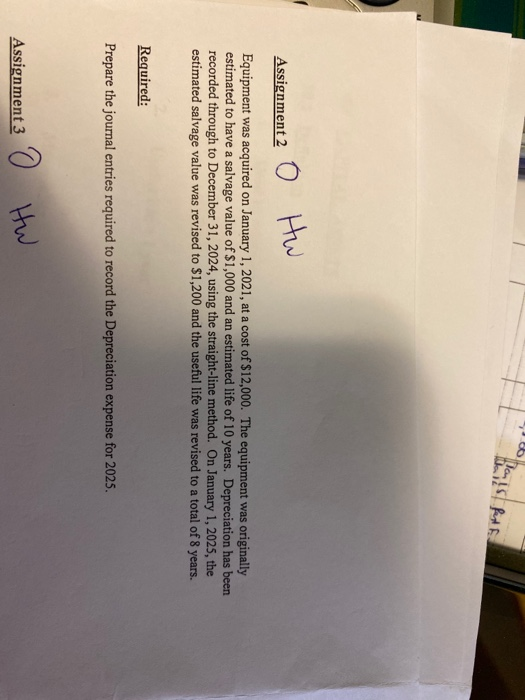Please check if it's correct. If not, please explain and re-do it. Thanks Assignment 2 O Hw Equipment was acquired on January 1, 2021, at a cost of \$12,000. The equipment was originally estimated to have a salvage value of \$1,000 and an estimated life of 10 years. Depreciation has been recorded through to December 31, 2024, using the straight-line method. On January 1, 2025, the estimated salvage value was revised to \$1,200 and the useful life was revised to...

• ### Depreciation Calcuations Woods ABO Fall 2019 Name: Jol moldrup Class: _10.30 Depreciation Components and Calculations Homework...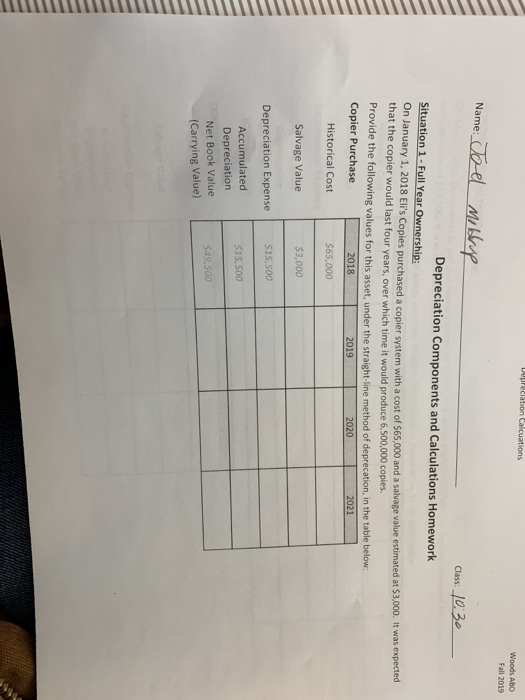Depreciation Calcuations Woods ABO Fall 2019 Name: Jol moldrup Class: _10.30 Depreciation Components and Calculations Homework Situation 1 - Full Year Ownership: on January 1, 2018 Eli's Copies purchased a copier system with a cost of \$65,000 and a salvage value estimated at 53,000. It was expected that the copier would last four years, over which time it would produce 6,500,000 copies Provide the following values for this asset, under the straight-line method of deprecation, in the table below Copier...

• ### 28) When originally purchased, a vehicle costing \$26.460 had an estimated useful life of 8 years...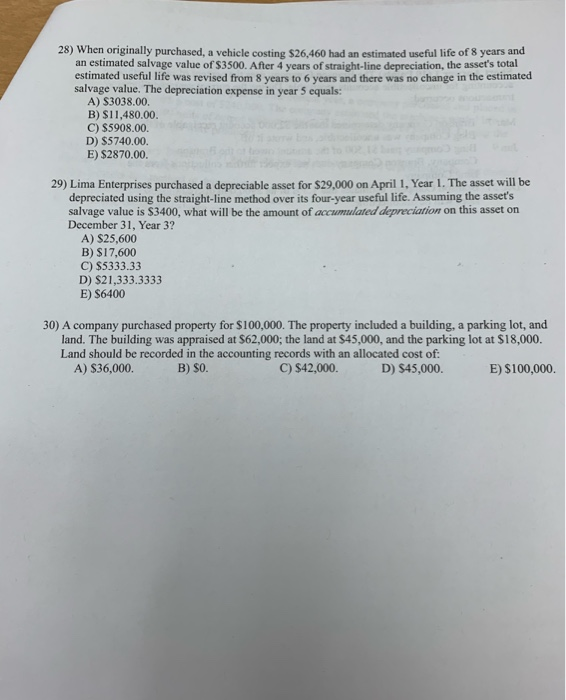28) When originally purchased, a vehicle costing \$26.460 had an estimated useful life of 8 years and an estimated salvage value of \$3500. After 4 years of straight-line depreciation, the asset's total estimated useful life was revised from 8 years to 6 years and there was no change in the estimated salvage value. The depreciation expense in year 5 equals: A) S3038.00. B) \$11,480.00. C) \$5908.00. D) \$5740.00 E) \$2870.00. 29) Lima Enterprises purchased a depreciable asset for \$29.000 on...

• ### how do you cakcukate it oncept Overview Videos Saved Help Save & Exit Submit Required information...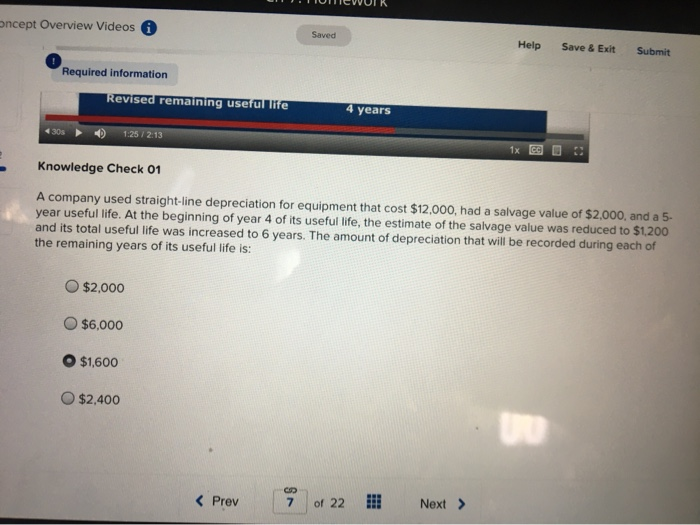how do you cakcukate it oncept Overview Videos Saved Help Save & Exit Submit Required information Revised remaining useful life 4 years 1305 1:25 / 2:13 1x CO Knowledge Check 01 A company used straight-line depreciation for equipment that cost \$12,000, had a salvage value of \$2,000, and a 5- year useful life. At the beginning of year 4 of its useful life, the estimate of the salvage value was reduced to \$1,200 and its total useful life was increased...

• ### \$19,000 \$15,000. \$4,000. \$34,000. D Question 10 If the straight-line method of depreciation of an asset...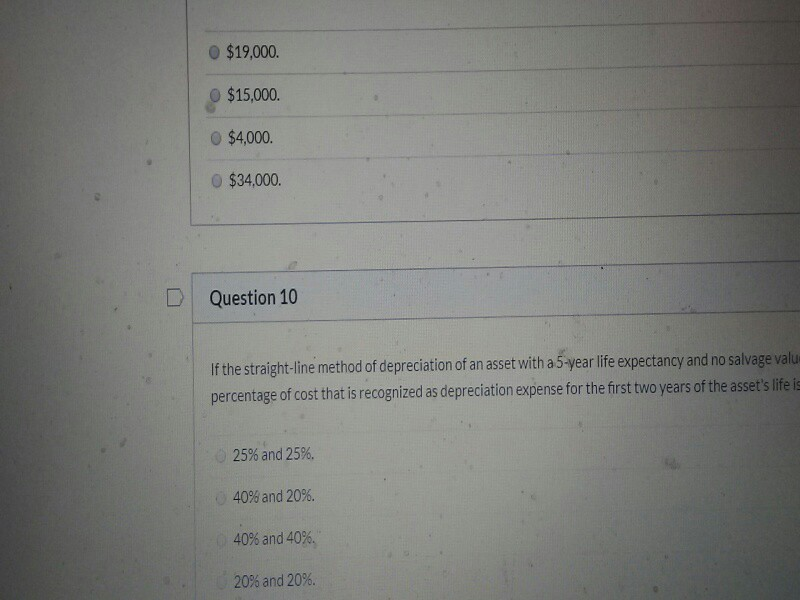\$19,000 \$15,000. \$4,000. \$34,000. D Question 10 If the straight-line method of depreciation of an asset with a 5-year life expectancy and no salvage valu percentage of cost that is recognized as depreciation expense for the first two years of the asset's lifell 25% and 25%. 40% and 20%. 40% and 40%. 20% and 20%.

Free Homework App